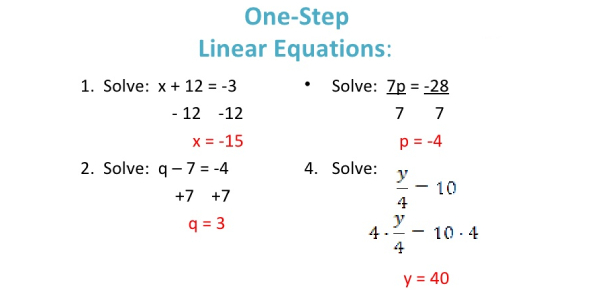# One Step Linear Equations Quiz! Math Test

25 Questions | Total Attempts: 133Settings.

Related Topics
• 1.
What is the inverse operation for multiplication?
• A.

• B.

Subtraction

• C.

Division

• 2.
What is the inverse operation for an addition?
• A.

Subtraction

• B.

Multiplication

• C.

Division

• 3.
What is the inverse operation for division?
• A.

• B.

Subtraction

• C.

Multiplication

• 4.
What is the inverse operation for subtraction?
• A.

• B.

Multiplication

• C.

Division

• 5.
6n = 30
• A.

5

• B.

-5

• C.

24

• D.

180

• 6.
9 = x / 18
• A.

-162

• B.

2

• C.

162

• D.

11

• 7.
X + 8 = 15
• A.

X = 7

• B.

X = -7

• C.

X = 23

• D.

X = -23

• 8.
Y - 3 = 5
• A.

Y = 8

• B.

Y = 15

• C.

Y = 2

• D.

Y = -2

• 9.
B + 2 = 19
• A.

B = 5

• B.

B = 17

• C.

B = 38

• D.

B = -5

• 10.
3x = 12
• A.

X = -4

• B.

X = 4

• C.

X = 36

• D.

X = 15

• 11.
2x = 88
• A.

X = 44

• B.

X = 86

• C.

X = -176

• D.

X = 176

• 12.
M + 43 = 100
• A.

M = 57

• B.

M = 143

• C.

M = -143

• D.

M = -57

• 13.
H - 34 = 17
• A.

H = 17

• B.

H = 51

• C.

H = -17

• D.

H = -51

• 14.
7d = 56
• A.

D = -8

• B.

D = 7

• C.

D = 8

• D.

D = 56

• 15.
R / 5 = 10
• A.

R = 2

• B.

R = 50

• C.

R = 5

• D.

R = 15

• 16.
Y / 12 = 11
• A.

Y = -132

• B.

Y = 12

• C.

Y = 1

• D.

Y = 132

• 17.
15 = x / 4
• A.

3.75

• B.

60

• C.

-11

• D.

-19

• 18.
• A.

198

• B.

-22

• C.

22

• D.

69

• 19.
40 = 10 + m
• A.

4

• B.

30

• C.

-30

• D.

0

• 20.
9 + x = 2
• A.

7

• B.

-11

• C.

-7

• D.

11

• 21.
5 = -20 + x
• A.

-15

• B.

25

• C.

100

• D.

15

• 22.
12 = x / 3
• A.

4

• B.

15

• C.

-4

• D.

36

• 23.
11x = 66
• A.

6

• B.

55

• C.

-297

• D.

-26

• 24.
5 = x / 12
• A.

50

• B.

60

• C.

2.5

• D.

17

• 25.
-3 + x = -12
• A.

-15

• B.

15

• C.

-9

• D.

9